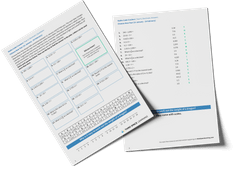Math teaching support you can trustone-on-one tutoring sessionsschools supported

[FREE] Fun Math Games & Activities Packs

Always on the lookout for fun math games and activities in the classroom? Try our ready-to-go printable packs for students to complete independently or with a partner!

# The Fraction Bar Model: How To Teach Fractions Using Bar Models

The fraction bar model is a great way to introduce fractions to your young math learners. Fractions can be a tricky concept for students to grasp and the visual representations of the bar model method can really help.

### What is the fraction bar model?

What is a bar model and how can it be used to teach fractions? Bar models, also known as strip diagrams, are part of the concrete pictorial abstract approach of teaching mathematical concepts and are an essential step in understanding the meaning of fractions and fraction concepts.

The fraction bar model uses a whole bar broken up into equal parts, a part whole model, to illustrate fractions of a whole. For example, a whole bar broken up into eight equal parts would be used to demonstrate eighths, and that eight eighths make up one whole.

A fraction wall uses a series of bar models to illustrate fractions and how different fractions relate to a whole.

### When do children learn to use the fraction bar model?

According to the Common Core and Texas standards for math, children will begin learning simple fractions, such as halves and quarters in 2nd and 3rd grade. As children progress in elementary school into 4th grade and 5th grade, they will be introduced to increasingly complex math problems using fractions where a bar model could help.

### Why teach bar model fractions?

Using the bar model is a great way to support childrens’ understanding of fractions and problem solving. Bar models can be used to teach both unit fractions and non-unit fractions. Bar models help children to visualize the math in front of them, organize their thoughts and solve problems.

Using the bar model can also be used to teach number bonds, place value,decimals, addition, subtraction, percentage, solve word problems and for bar model multiplication and division.

### How to draw a fraction bar model

Drawing a fraction bar model is simple:

• Draw a rectangle, it does not matter what size.
• Look at the denominator of the fraction you wish to represent.
• Divide the rectangle into the same number of equal parts as the denominator of the fraction.
• Shade in the same number of pieces as the numerator of the fraction.

### Finding fractions using the fraction bar model

Using the bar model can be used to help children find fractions of an amount, for example ⅕ of 25. Instead of starting with the fraction, students start with the whole number which they then must divide into fifths.

The bar model helps to illustrate that five equal parts are added together to make the whole.

### Improper fractions and the fraction bar model

Improper fractions are fractions that have a larger numerator than the denominator. Children will often be asked to convert improper fractions into mixed numbers. In this case, using the bar model can be very useful.

A mixed number is a whole number combined with a fraction, for example, 3 ⅔.

This mixed number and its equivalent improper fraction can be represented using the fraction bar model.

### Equivalent fractions and the fraction bar model

Some math problems may require children to identify equivalent fractions. Bar models can make this a lot easier as it removes the abstract and instead uses a concrete visualization, enabling children to compare visuals and identify equivalent fractions.

### More fractions and bar models resources

Third Space Learning’s math hub provides a wide range of elementary school resources such as printable worksheets, for teaching fractions and bar models. Our online one-to-one tuition also uses bar models to help children to gain a deep understanding of mathematical concepts.

Do you have students who need extra support in math?
Give your students more opportunities to consolidate learning and practice skills through personalized math tutoring with their own dedicated online math tutor.

Each student receives differentiated instruction designed to close their individual learning gaps, and scaffolded learning ensures every student learns at the right pace. Lessons are aligned with your state’s standards and assessments, plus you’ll receive regular reports every step of the way.

Personalized one-on-one math tutoring programs are available for:

The content in this article was originally written by content team Vanessa Sipple-Asher and has since been revised and adapted for US schools by elementary math teacher Jaclyn Wassell.##### Vanessa Sipple-Asher
Third Space Learning
Content Team
Vanessa edits a lot of the content that you see on the Third Space Learning blog and once in a while, she gets the chance to write articles about maths education and how Third Space Learning can help students and teachers.
xx

#### [FREE] Fun Math Games & Activities Packs

Individual packs containing fun math games and activities to complete in the classroom.

The activities are designed to be fun, flexible and suitable for a range of abilities.